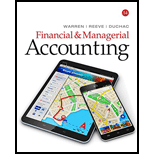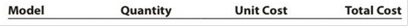# Periodic inventory by three methods Pappa’s Appliances uses the periodic inventory system. Details regarding the inventory of appliances at January 1, purchases invoices during the year, and the inventory count at December 31 are summarized as follows: Purchases Invoices Model Inventory, January 1 1 st 2nd 3rd Inventory Count, December 31 C55 3 at $1,040 3 at$1,054 3 at $1,060 3 at$1,070 4 D11 9at 639 7 at 645 6 at 666 6at 675 11 F32 5 at 240 3 at 260 1 at 260 1 at 280 2 H29 6 at 305 3 at 310 3 at 316 4 at 317 4 K47 6at 520 8 at 531 4at 549 6 at 542 8 Instructions 1. Determine the cost of the inventory on December 31 by the first-in, first-out method. Present data in columnar form, using the following headings: If the inventory of a particular model comprises one entire purchase plus a portion of an-other purchase acquired at a different unit cost, use a separate line for each purchase. 2. Determine the cost of the inventory on December 31 by the last-in, first-out method, following the procedures indicated in (1). 3. Determine the cost of the inventory on December 31 by the weighted average cost method, using the columnar headings indicated in (1). 4. Discuss which method (FIFO or LIFO) would be preferred for income tax purposes in periods of (A) rising prices and (B) declining prices.### Financial & Managerial Accounting

14th Edition
Carl Warren + 2 others
Publisher: Cengage Learning
ISBN: 9781337119207

#### Solutions

Chapter
Section### Financial & Managerial Accounting

14th Edition
Carl Warren + 2 others
Publisher: Cengage Learning
ISBN: 9781337119207
Chapter 6, Problem 6.5BPR
Textbook Problem
16 views

## Periodic inventory by three methodsPappa’s Appliances uses the periodic inventory system. Details regarding the inventory of appliances at January 1, purchases invoices during the year, and the inventory count at December 31 are summarized as follows:     Purchases Invoices   Model Inventory, January 1 1 st 2nd 3rd Inventory Count, December 31 C55 3 at $1,040 3 at$1,054 3 at $1,060 3 at$1,070 4 D11 9at 639 7 at 645 6 at 666 6at 675 11 F32 5 at 240 3 at 260 1 at 260 1 at 280 2 H29 6 at 305 3 at 310 3 at 316 4 at 317 4 K47 6at 520 8 at 531 4at 549 6 at 542 8 Instructions 1. Determine the cost of the inventory on December 31 by the first-in, first-out method. Present data in columnar form, using the following headings:If the inventory of a particular model comprises one entire purchase plus a portion of an-other purchase acquired at a different unit cost, use a separate line for each purchase. 2. Determine the cost of the inventory on December 31 by the last-in, first-out method, following the procedures indicated in (1). 3. Determine the cost of the inventory on December 31 by the weighted average cost method, using the columnar headings indicated in (1). 4. Discuss which method (FIFO or LIFO) would be preferred for income tax purposes in periods of (A) rising prices and (B) declining prices.

(1)

To determine

Periodic Inventory System:

Periodic inventory system is a system, in which the inventory is updated in the accounting records on a periodic basis such as at the end of each month, quarter or year. In other words, it is an accounting method which is used to determine the amount of inventory at the end of each accounting period.

First-in-First-Out:

In First-in-First-Out method, the costs of the initially purchased items are considered as cost of goods sold, for the items which are sold first. The value of the ending inventory consists of the recent purchased items.

Last-in-Last-Out:

In Last-in-First-Out method, the costs of last purchased items are considered as the cost of goods sold, for the items which are sold first. The value of the closing stock consists of the initial purchased items.

Weighted-average cost method:

Under Weighted average cost method, the company calculates a new average cost after every purchase is made. It is determined by dividing the cost of goods available for sale by the units on hand.

To determine: value of inventory using first in first out method under periodic inventory system.

### Explanation of Solution

The tabular column showing inventory cost is presented as follows:

 Model Quantity ($) Unit cost ($) Total cost (\$) C55 3 1,070 3,210 1 1,060 1,060 D11 6 675 4,050 5

(2)

To determine
value of inventory using last in first out method under periodic inventory system.

(3)

To determine
value of inventory using weighted average method under periodic inventory system.

4

(a)

To determine

To discuss: the method that would be preferred for income tax purposes in the period of rising prices.

### Still sussing out bartleby?

Check out a sample textbook solution.

See a sample solution

#### The Solution to Your Study Problems

Bartleby provides explanations to thousands of textbook problems written by our experts, many with advanced degrees!

Get Started

Find more solutions based on key concepts
How is organization height related to the span of management?

Foundations of Business (MindTap Course List)

Distinguish between exposure and risk.

Accounting Information Systems

PREFERRED STOCK VALUATION Ezzell Corporation issued perpetual preferred stock with a 10% annual dividend. The s...

Fundamentals of Financial Management, Concise Edition (with Thomson ONE - Business School Edition, 1 term (6 months) Printed Access Card) (MindTap Course List)